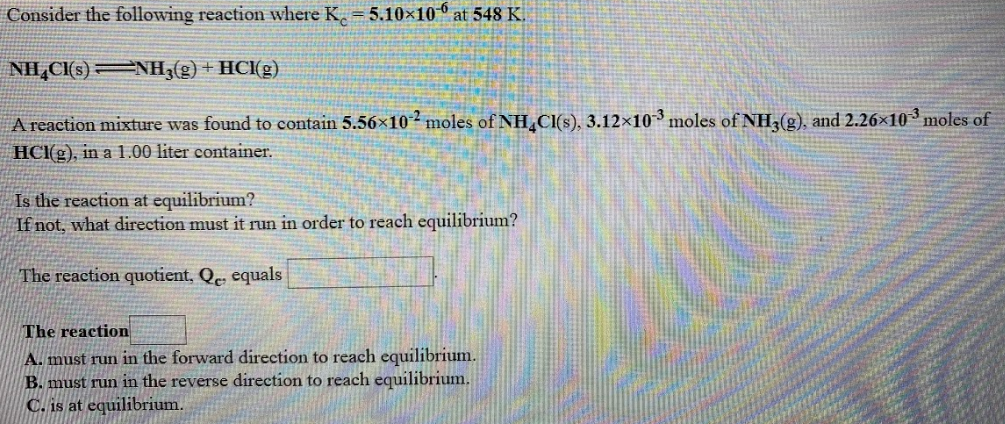# Consider the following reaction where Kc = 5.10x10^-6 at 548 K. NH4Cl(s) ⇌ NH3(g) + HCl(g) A reaction mixture was found to contain 5.56x10^-2 moles of NH4Cl(s), 3.12x10^-3 moles of NH3(g), and 2.26x10^-3 moles of HCl(g), in a 1.00 liter container. Is the reaction at equilibrium? If not, what direction must it run in order to reach equilibrium? The reaction quotient, Qc, equals ____. The reaction ____.